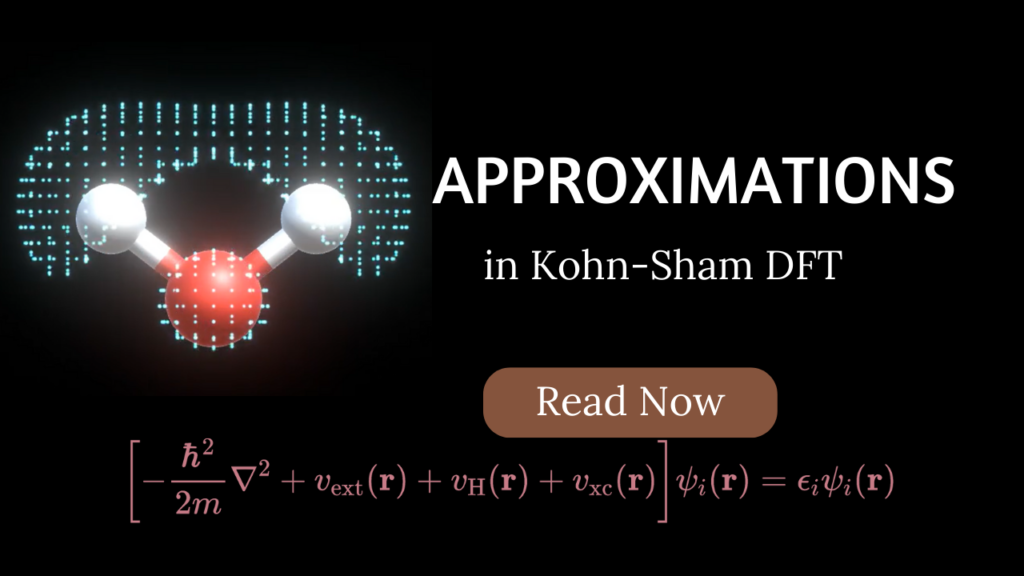# What are the approximations in Kohn-Sham DFT?In Kohn-Sham density functional theory (KS-DFT), the ground state electron density is described using a single mathematical determinant, which leads to a set of equations that are similar to those used in Hartree-Fock theory and are known as Kohn-Sham equations:$\left[-\frac{\hbar^2}{2m}\nabla^2 + v_{\text{ext}}(\mathbf{r}) + v_{\text{H}}(\mathbf{r}) + v_{\text{xc}}(\mathbf{r})\right] \psi_i(\mathbf{r}) = \epsilon_i \psi_i(\mathbf{r})$

where$v_{\text{ext}}(\mathbf{r})$ is the external potential (such as the potential from the nuclei),$v_{\text{H}}(\mathbf{r})$ is the Hartree potential (which describes the electrostatic interaction between the electrons), and$v_{\text{xc}}(\mathbf{r})$ is the exchange-correlation potential (which describes the many-body effects in the system). The wavefunction$\psi_i(\mathbf{r})$ (KS orbitals) and energy$\epsilon_i$ are the solutions to the Kohn-Sham equation for the$i$th electron.

The total energy is composed of the non-interacting kinetic energy$T_s$, the energy due to the electrostatic interactions of the electrons with their charge density and with the nuclear cores$E_{elecstatic}$, and the exchange-correlation energy$E_{XC}$ that accounts for the remaining electronic energy not included in the non-interacting kinetic and electrostatic terms like the non-classical electron-electron repulsion and the difference between interacting and non-interacting kinetic energy$T_c[\rho] = T[\rho]-T_s[\rho]$.$E[\rho] = T_s + E_{elecstatic}[\rho] + E_{XC}[\rho]$

### Approximations

Here are some of the main approximations used in Kohn-Sham DFT:

•  In KS-DFT, one avoids the need for a kinetic energy functional$T[\rho]$ by evaluating the exact kinetic energy$T_s=-\frac{1}{2} \sum_{i=1}^N \int \psi_i^*(\boldsymbol{r}) \nabla^2 \psi_i(\boldsymbol{r}) d \boldsymbol{r}$ from the wavefunction (single Slater determinant) of a fictitious non-interacting system with the same density as that of the real one. Therefore, KS-DFT calculates the kinetic energy in terms of orbitals. However, one still needs to approximate the$T_c[\rho]$ (mentioned above). Since we are again working with orbitals in KS-DFT (like in the case of Hartree-Fock theory), we need a set of basis functions (atomic orbitals) for each atom of our system whose linear combination corresponds to KS orbitals (molecular orbitals). This set of basis functions is called a basis set and makes the calculation accuracy dependent on the quality of basis sets used.
• Use of the exchange-correlation functional, which approximates the exchange and correlation energy of a many-electron system. The exchange-correlation energy accounts for the remaining electronic energy not included in the non-interacting kinetic and electrostatic terms. Some of the common types are: local (LDA), semi-local (GGA), and hybrid (including some portion of exact exchange) functionals that differ in accuracy.
• Approximate exchange-correlation functionals also lead to self-interaction errors (SEI) which in turn lead to delocalization errors. In both HF theory and KS-DFT, the potential field includes the Coulomb potential, which is the interaction of the electron with the entire electron density of the atom, molecule, or material. That is physically incorrect because an electron does not interact with itself. In HF theory, however, the exchange potential (non-local in nature) completely cancels the self-interaction part of the Coulomb potential which is not the case for KS-DFT, due to the approximate (not completely non-local) exchange-correlation functionals. It has been known to be the primary cause for the underestimation of band-gaps in semiconductors.
• Ignoring relativistic effects, which can be important for certain types of systems. Standard DFT does not take into account relativistic effects like spin-orbit coupling. However, many codes have the ability to account for it by using relativistic pseudopotentials.
• Use of Born-Oppenheimer approximation. The positions of the nuclear cores are considered parameters of the electronic Hamiltonian thus defining a potential energy surface. The electronic system is assumed to adiabatically follow changes in the nuclear coordinates relaxing to its ground state on much faster timescales than the timescales of nuclear motion (electron-phonon coupling, e.g., is a correction to this adiabatic approximation).

If you have any questions/doubts or find any mistake in the above article then please let me know in the comments section down below.

### Other useful resources:

What is the difference between DFT and Hartree-Fock method?

[wpedon id="7041" align="center"]

This site uses Akismet to reduce spam. Learn how your comment data is processed.